## NONLINEAR DYNAMIC ANALYSIS OF A VAN DER POL-MATHIEU EQUATION WITH EXTERNAL EXCITATION1)

Huang Jianliang,2), Wang Teng, Chen Shuhui

Department of Applied Mechanics and Engineering, Sun Yat-sen University, Guangzhou 510275, China

 基金资助: 1) 国家自然科学基金资助项目.  11972381

Received: 2020-09-6   Accepted: 2020-11-2   Online: 2021-02-07Abstract

The periodic responses and quasi-periodic motions of a van der Pol-Mathieu equation subjected to three excitations, i.e., self-excited, parametric excitation, and external excitation, are studied in this paper. A new characteristic is observed that the spectra of the quasi-periodic motions contain uniformly spaced sideband frequencies. Firstly, the traditional incremental harmonic balance (IHB) method is used to obtain periodic responses of the van der Pol-Mathieu equation and to trace their nonlinear frequency response curves automaically. Then the Floquet theory is used to analyze stability of the periodic responses and their bifurcations. Based on the characteristic that the spectra of quasi-periodic motions contain two incommensurate basic frequencies, i.e., the excitation frequency and a priori unknown frequency related to uniformly spaced sideband frequencies. Then the IHB method with two time-scales basing on the two basic frequencies is formulated to accurately calculate all frequency components and their corresponding amplitudes even at critical points. All the results obtained from the IHB method with two time-scales are in excellent agreement with those from numerical integration using the fourth-order Runge-Kutta method. Finally, this investigation reveals rich dynamic characteristics of the van der Pol-Mathieu equation in a range of excitation frequencies.

Keywords： van der Pol-Mathieu equation ; incremental harmonic balance method with two time-scales ; bifurcation ; quasi-periodic motion ; sideband

Huang Jianliang, Wang Teng, Chen Shuhui. NONLINEAR DYNAMIC ANALYSIS OF A VAN DER POL-MATHIEU EQUATION WITH EXTERNAL EXCITATION1). Chinese Journal of Theoretical and Applied Mechanics[J], 2021, 53(2): 496-510 DOI:10.6052/0459-1879-20-310

## 引言

Tondl首先分析了van der Pol-Mathieu方程中自激振动和参数激振的相互作用, 并在共振区域发现了周期响应. Kotera和Yano用两个频率的和分析了van der Pol-Mathieu方程在参数共振区域的近似一阶和二阶的周期解. 陈予恕和徐鉴研究了van der Pol-Duffing-Mathieu型系统主参数共振分岔解, 得到该非线性参数激励系统依赖于物理参数变化的振动模式. Szabelski和Warminski分析了自激振动和参数激励对van der Pol-Mathieu方程的影响, 并且研究了附加外激励在同步区域内动力学行为的影响. Warminski等对含有自激振动和参数激励的两自由度系统进行分析, 并得到了不同类型的响应, 包含有周期响应, 准周期运动响应和混沌. 彭献和陈自力引入参数变换, 将强非线性系统转化为弱非线性系统, 利用摄动思想分析得到了van der Pol-Mathieu方程的1/2亚谐共振周期解. Belhaq和Fahsi和Pandey等分析了van der Pol-Mathieu-Duffing系统的响应, 得到该类系统可含有1:1锁频, 2:1次谐波锁频和准周期运动响应. 张琪昌等利用改进的类Padé方法计算了van der Pol-Duffing方程的同异宿轨道. Warminski研究了含有van der Pol和Rayleigh函数在两个不同自激振动模型下具有时滞状态的自激振动, 参数激振和强迫振动作用下的相互作用. 许多学者也对各类含有参数激振的非线性系统进行研究[16-19], 得到了不同的非线性振动特性和运动分岔.

## 1 含外激励van der Pol-Mathieu方程的周期解及其稳定性

$\begin{eqnarray} &&\frac{{\rm d}^2x}{{\rm d}t^2}-\left(k_1-k_2x^2\right)\frac{{\rm d}x}{{\rm d}t}+\left(\tilde{\omega}_0^2+k_3\cos{2\omega t}\right)x=\\&&\qquad f\cos\omega t \label{eq1} \end{eqnarray}$

### 1.1 传统的IHB法

$\begin{eqnarray} \tau=\omega t \label{eq2} \end{eqnarray}$

$\begin{eqnarray} &&\omega^2x"-\omega\left(k_1-k_2x^2\right)x'+\left(\tilde{\omega}_0^2+k_3\cos2\tau\right)x=\\&&\qquad f\cos\tau \label{eq3} \end{eqnarray}$

$\begin{eqnarray} x=x_0+\Delta x, \ \ \omega=\omega_0+\Delta\omega, \ \ f=f_0+\Delta f \label{eq4} \end{eqnarray}$

$\begin{eqnarray} &&\omega_0^2 \Delta x"-\omega_0\left(k_1-k_2x^2_0\right)\Delta x' +\\&&\qquad\left(\tilde{\omega}_0^2+k_3\cos2\tau+2\omega_0k_2x_0x_0'\right)\Delta x =\\&&\qquad R-\left[2\omega_0x_0"-\left(k_1-k_2x_0^2\right)\right]\Delta\omega+\cos\tau\Delta f \label{eq5} \end{eqnarray}$

$\begin{eqnarray} &&R=f_0\cos\tau -\Big[\omega_0^2x"_0 -\omega_0\left(k_1-k_2x_0^2\right)x'_0 +\\&&\qquad\left(\tilde{\omega}_0^2+k_3\cos2\tau\right)x_0\Big] \label{eq6} \end{eqnarray}$

$\begin{eqnarray} &&x_0=\sum_{k=1}^{n_{\rm c}}a_k\cos\left(2k-1\right)\tau+\\&&\qquad \sum_{k=1}^{n_{\rm s}}b_k\sin\left(2k-1\right)\tau=\boldsymbol C _{\rm s}\boldsymbol A \label{eq7} \end{eqnarray}$
$\begin{eqnarray} \Delta x=\sum_{k=1}^{n_{\rm c}}\Delta a_k\cos\left(2k-1\right)\tau+\\ \qquad\sum_{k=1}^{n_{\rm s}}\Delta b_k\sin\left(2k-1\right)\tau =\boldsymbol C_{\rm s}\Delta\boldsymbol A \label{eq8} \end{eqnarray}$

$\begin{eqnarray} \boldsymbol C_{\rm s}= [\cos\tau \ \cos3\tau \ \cdots \ \cos\left(2n_{\rm c}-1\right)\tau \\ \qquad \sin\tau \ \sin3\tau \ \cdots \ \sin \left(2n_{\rm s}-1\right) ] \end{eqnarray}$
$\begin{eqnarray} \boldsymbol A= \begin{bmatrix} a_1 & a_2 & \cdots & a_{n_{\rm c}} & b_1 & b_2 & \cdots & b_{n_{\rm s}} \end{bmatrix} ^{\rm T} \label{eq10} \end{eqnarray}$
$\begin{eqnarray} \Delta \boldsymbol A= \begin{bmatrix}\Delta a_1 & \Delta a_2 & \cdots & \Delta a_{n_{\rm c}} & \Delta b_1 & \Delta b_2 & \cdots & \Delta b_{n_{\rm s}} \end{bmatrix} ^{\rm T} \label{eq11} \end{eqnarray}$

$\begin{eqnarray} &&x'_0=\boldsymbol C'_{\rm s}\boldsymbol A, \ \ \Delta x'=\boldsymbol C'_{\rm s}\Delta\boldsymbol A \label{eq12} \end{eqnarray}$
$\begin{eqnarray} x"_0=\boldsymbol C"_{\rm s}\boldsymbol A, \ \ \Delta x"=\boldsymbol C"_{\rm s}\Delta\boldsymbol A \label{eq13} \end{eqnarray}$

$\begin{eqnarray}\label{eq14} &&\int_{0}^{2\pi} \delta \left(\Delta x\right)\Big[\omega_0^2 \Delta x"-\omega_0\left(k_1-k_2x_0^2\right)\Delta x'+\\&&\qquad\left(\tilde{\omega}_0 ^2+k_3\cos2\tau+2\omega_0k_2x_0x'_0\right)\Delta x \Big]{\rm d}\tau =\\&&\qquad \int_{0}^{2\pi}\delta\left(\Delta x\right)\Big\{ R-\left[2\omega_0x"_0-\left(k_1-k_2x_0^2\right)x'_0\right]\Delta\omega +\\&&\qquad \cos\tau\Delta f\Big\}{\rm d}\tau \end{eqnarray}$

$\begin{eqnarray} \boldsymbol K_A\Delta \boldsymbol A=\bar{\boldsymbol R}-\boldsymbol R_\omega \Delta \omega+\boldsymbol R_f\Delta f \label{eq15} \end{eqnarray}$

$\begin{eqnarray} \boldsymbol K_A=\int_{0}^{2\pi}\boldsymbol C_{\rm s}^{\rm T}\Big[\omega_0^2\boldsymbol C"_{\rm s}-\omega_0\left(k_1-k_2\boldsymbol C_{\rm s}\boldsymbol A\boldsymbol C_{\rm s}\boldsymbol A\right)\boldsymbol C'_{\rm s}+\\ \qquad \left(\tilde{\omega}_0^2+k_3\cos2\tau+2\omega_0k_2\boldsymbol{C}_{\rm s}\boldsymbol{A}\boldsymbol{C}'_{\rm s}\boldsymbol{A}\right)\Big]{\rm d}\tau \label{eq16} \end{eqnarray}$
$\begin{eqnarray} \bar{\boldsymbol{R}}=-\int_{0}^{2\pi}\boldsymbol{C}_{\rm s}^{\rm T}\Big[\omega_0^2\boldsymbol{C}"_{\rm s}-\omega_0\left(k_1-k_2\boldsymbol{C}_{\rm s}\boldsymbol{A}\boldsymbol{C}_{\rm s}\boldsymbol{A}\right)\boldsymbol{C}'_{\rm s}+\\ \qquad\left(\tilde{\omega}_0^2+k_3\cos2\tau\right)\Big]{\rm d}\tau \label{eq17} \end{eqnarray}$
$\boldsymbol{R}_{\omega}=\int_{0}^{2 \pi} \boldsymbol{C}_{\mathrm{s}}^{\mathrm{T}}\left[2 \omega_{0} \boldsymbol{C}_{\mathrm{s}}^{\prime \prime}-\left(k_{1}-k_{2} \boldsymbol{C}_{\mathrm{s}} \boldsymbol{A} \boldsymbol{C}_{\mathrm{s}} \boldsymbol{A}\right) \boldsymbol{C}_{\mathrm{s}}^{\prime}\right] \boldsymbol{A} \mathrm{d} \tau$
$\begin{eqnarray} \boldsymbol{R}_f=\int_{0}^{2\pi}\boldsymbol{C}'_{\rm s}\cos\tau {\rm d}\tau \label{eq19} \end{eqnarray}$

$\begin{eqnarray} \boldsymbol{K}_A\Delta \boldsymbol{A}=\bar{\boldsymbol{R}}-\boldsymbol{R}_\omega \Delta \omega\label{eq20} \end{eqnarray}$

### 1.2 周期解的稳定性与分岔

$\begin{eqnarray} x=x_0+\Delta x\label{eq21} \end{eqnarray}$

$\begin{eqnarray} && \omega^2\Delta x"-\omega \left(k_1-k_2x_0^2\right)\Delta x' +\\&&\qquad \left(\tilde{\omega}_0^2+k_3\cos2\tau+2k_2x_0x'_0\right)\Delta x=0 \label{eq22} \end{eqnarray}$

$\begin{eqnarray} \boldsymbol{X}'=\boldsymbol{Q}\left(\tau\right)\boldsymbol{X} \label{eq23} \end{eqnarray}$

$\begin{eqnarray} &&\boldsymbol{X}=\begin{bmatrix} \Delta x & \Delta x' \end{bmatrix}^{\rm T} \label{eq24} \\ \end{eqnarray}$
$\begin{eqnarray} &&\boldsymbol{Q}=\begin{bmatrix} 0 & 1 \\ Q_{21}\left(\tau\right) & Q_{22}\left(\tau\right) \end{bmatrix} \label{eq25} \end{eqnarray}$

$\begin{eqnarray} &&Q_{21}\left(\tau\right)=-\frac{1}{\omega^2}\left(\tilde{\omega}_0^2+k_3\cos2\tau+2k_2x_0x'_0\right) \label{eq26} \end{eqnarray}$
$\begin{eqnarray} &&Q_{22}\left(\tau\right)=\frac{1}{\omega}\left(k_1-k_2x_0^2\right) \label{eq27} \end{eqnarray}$

$\begin{eqnarray} \boldsymbol{y}_k=\begin{bmatrix} y_{1k} & y_{2k} \end{bmatrix},\ \ k=1,2 \label{eq28} \end{eqnarray}$

$\begin{eqnarray} \boldsymbol{Y}= \begin{bmatrix} y_{11} & y_{12} \\ y_{21} & y_{22} \end{bmatrix} \label{eq29} \end{eqnarray}$

$\begin{eqnarray} \boldsymbol{Y}'=\boldsymbol{Q}\left(\tau\right)\boldsymbol{Y} \label{eq30} \end{eqnarray}$

$\begin{eqnarray} \boldsymbol{Y}\left(\tau+T\right)=\boldsymbol{PY}\left(\tau\right)\label{eq31} \end{eqnarray}$

### 1.3 含外激励van der Pol-Mathieu方程周期解的响应特性

$\begin{eqnarray} &&x_0=a_1\cos\tau+a_2\cos 3\tau+a_3\cos 5\tau+b_1\sin\tau+\\&&\qquad b_2\sin 3\tau+ b_3\sin 5\tau =A_1\cos\left(\tau+\varphi_1\right)+\\&&\qquad A_2\cos\left(3\tau+\varphi_2\right)+ A_3\cos\left(5\tau+\varphi_3\right) \label{eq32} \end{eqnarray}$

$\begin{eqnarray} A_i=\sqrt{a_i^2+b_i^2}, \ \ \varphi_i=\arctan\left(-b_i/a_i\right), \ \ i=1,2,3\quad \label{eq33} \end{eqnarray}$

### 图1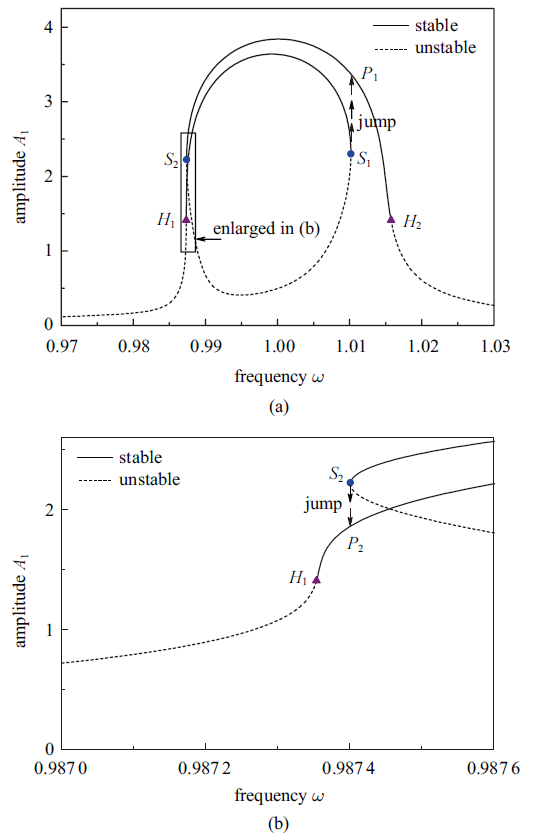Fig.1   Frequency response curve $\omega$-$A_1$ of periodic response of the van der Pol-Mathieu equation with external excitation with $k_1=0.01$, $k_2=0.01$, $k_3=0.05$, and $f=0.01$, (b) is an enlarged view of a zone highlighted in (a)

Table 1  Floquet multipliers $\lambda_1$ and $\lambda_2$ for the frequency $\omega$ near the four bifurcation points $S_1$, $S_2$, $H_1$, and $H_2$, where $\bar{\rm i}=\sqrt{-1}$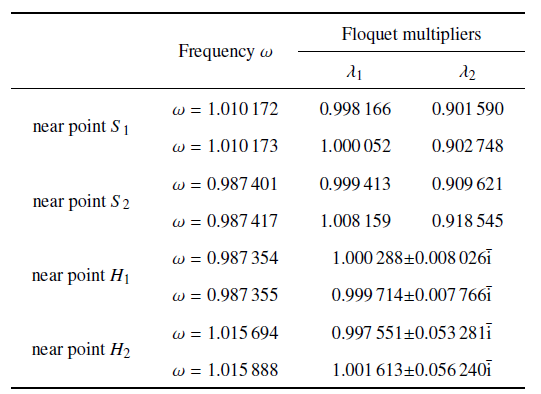### 图2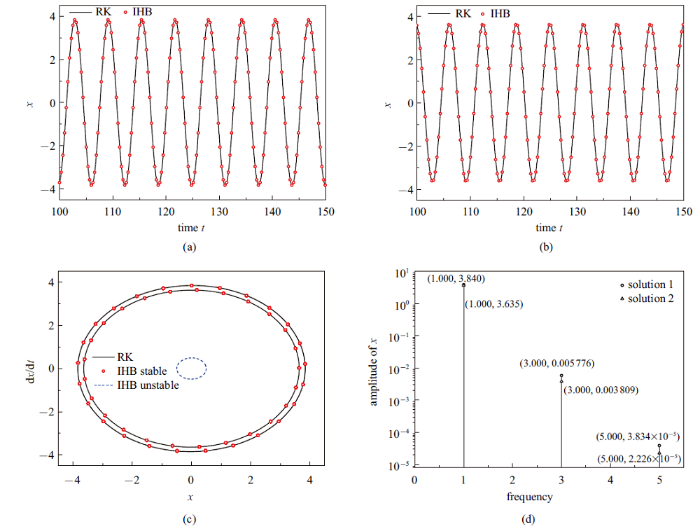Fig.2   Three different periodic solutions of the van der Pol-Mathieu equation with external excitation for $\omega=1.0$ with $k_1=0.01$, $k_2=0.01$, $k_3=0.05$, and $f=0.01$: (a) Time history of the first stable periodic response; (b) time history of the second stable periodic response; (c) phase plane portraits of the three solutions; (d) Fourier spectra of the two stable periodic response

## 2 含外激励van der Pol-Mathieu方程的准周期运动

### 2.1 两时间尺度的IHB法

(1) 第一点改进是引入两个时间变量代替式(2)中针对于周期解的一个时间变量$\tau=\omega t$, 如下

$\begin{eqnarray} \tau_1=\omega t, \ \ \tau_2=\omega_{\rm d}t \label{eq34} \end{eqnarray}$

$\left. \begin{array} \\ \Theta=\dfrac{\rm d}{{\rm d}t}=\omega\dfrac{\partial}{\partial \tau_1}+\omega_{\rm d}\dfrac{\partial}{\tau_2} \\[3mm]\Theta^2=\dfrac{{\rm d}^2}{{\rm d}^2t}=\omega^2\dfrac{\partial^2}{\partial\tau_1^2}+2\omega\omega_{\rm d}\dfrac{\partial^2}{\partial\tau_1\partial\tau_2}+\omega_{\rm d}^2\dfrac{\partial^2}{\partial\tau_2^2} \label{eq35} \\ \end{array} \right\}$

$\begin{eqnarray} &&\Theta^2x-\left(k_1-k_2x^2\right)\Theta x+\left(\tilde{\omega}_0^2+k_3\cos2\tau_1\right)x=\\&&\qquad f\cos\tau_1 \label{eq36} \end{eqnarray}$

$\left. \begin{array} \\ x=x_0+\Delta x, \ \ \omega=\omega_0+\Delta\omega\\ \omega_{\rm d}=\omega_{\rm d0}+\Delta\omega_{\rm d}, \ \ f=f_0+\Delta f \end{array} \right\}$

$\left. \begin{array} \\ \Theta_0=\omega_0\dfrac{\partial}{\partial \tau_1}+\omega_{\rm d0}\dfrac{\partial}{\tau_2} \\ \Theta_0^2=\omega_0^2\dfrac{\partial^2}{\partial\tau_1^2}+2\omega_0\omega_{\rm d0} \dfrac{\partial^2}{\partial\tau_1\partial\tau_2}+\omega_{\rm d0}^2\dfrac{\partial^2}{\partial\tau_2^2} \label{eq38} \\ \end{array} \right\}$

$\begin{array}{l}\Theta_{0}^{2} \Delta x-\left(k_{1}-k_{2} x_{0}^{2}\right) \Theta_{0} \Delta x+\left(\tilde{\omega}_{0}^{2}+k_{3} \cos 2 \tau_{1}+\right. \\\left.2 x_{0} \Theta_{0} x_{0}\right) \Delta x=\tilde{R}-\left[2\left(\omega_{0} \frac{\partial^{2} x_{0}}{\partial \tau_{1}^{2}}+\omega_{\mathrm{d} 0} \frac{\partial^{2} x_{0}}{\partial \tau_{1} \partial \tau_{2}}\right)-\right. \\\left.\left(k_{1}-k_{2} x^{2}\right) \frac{\partial x_{0}}{\partial \tau_{1}}\right] \Delta \omega-\left[2\left(\omega_{0} \frac{\partial^{2} x_{0}}{\partial \tau_{1} \partial \tau_{2}}+\omega_{\mathrm{d} 0} \frac{\partial^{2} x_{0}}{\partial \tau_{2}^{2}}\right)-\right. \\\left.\left(k_{1}-k_{2} x^{2}\right) \frac{\partial x_{0}}{\partial \tau_{2}}\right] \Delta \omega_{\mathrm{d}}+\cos \tau \Delta f\end{array}$

\begin{aligned}\tilde{R}=& f_{0} \cos \tau_{1}-\left[\Theta_{0}^{2} x_{0}-\left(k_{1}-k_{2} x^{2}\right) \Theta_{0} x_{0}+\right.\\&\left.\left(\tilde{\omega}_{0}^{2}+k_{3} \cos 2 \tau_{1}\right) x_{0}\right]\end{aligned}

(2) 第二点改进是将$x_0$展开为含有两个时间变量$\tau_1$和$\tau_2$的二重傅里叶级数形式

$\begin{eqnarray} &&x_0=\sum_{j_1=1}^{N_m}\sum_{j_2=-N_m}^{N_m}\tilde{a}_{j_1,j_2}\cos\left(j_1\tau_1+j_2\tau_2\right) +\\&&\qquad\sum_{j_1=1}^{N_m}\sum_{j_2=-N_m}^{N_m}\tilde{b}_{j_1,j_2}\sin\left(j_1\tau_1+j_2\tau_2\right) \label{eq41} \end{eqnarray}$

$\begin{eqnarray} &&x_0=\underbrace{\sum_{j_2=-m_1}^{m_1}\tilde{a}_{1,j_2}\cos\left(\tau_1+j_2\tau_2\right)}_{2m_1+1}+\\&&\qquad \underbrace{\sum_{j_2=-m_2}^{m_2}\tilde{a}_{3,j_2}\cos\left(3\tau_1+j_2\tau_2\right)}_{2m_2+1} +\cdots+\\&&\qquad\underbrace{\sum_{j_2=-m_{n_{\rm c}}}^{m_{n_{\rm c}}}\tilde{a}_{\left(2n_{\rm c}-1\right),j_2}\cos\left[\left(2n_{\rm c}-1\right)\tau_1+j_2\tau_2\right]}_{2m_{n_{\rm c}}+1} +\\&&\qquad\underbrace{\sum_{j_2=-m_1}^{m_1}\tilde{b}_{1,j_2}\sin\left(\tau_1+j_2\tau_2\right)}_{2m_1+1}+\\&&\qquad \underbrace{\sum_{j_2=-m_2}^{m_2}\tilde{b}_{3,j_2}\sin\left(3\tau_1+j_2\tau_2\right)}_{2m_2+1} +\cdots+\\&&\qquad\underbrace{\sum_{j_2=-m_{n_{\rm s}}}^{m_{n_{\rm s}}}\tilde{b}_{\left(2n_{\rm s}-1\right),j_2}\sin\left[\left(2n_{\rm s}-1\right)\tau_1+j_2\tau_2\right]}_\text{2m_{n_{\rm s}}+1}=\\&&\qquad \tilde{\boldsymbol{C}}_{\rm s}\tilde{\boldsymbol{A}} \end{eqnarray}$

\begin{eqnarray} &&\tilde{\boldsymbol{C}}_{\rm s}=\begin{bmatrix} \tilde{\boldsymbol{C}}_1 & \tilde{\boldsymbol{C}}_2 & \cdots & \tilde{\boldsymbol{C}}_{n_{\rm c}} & \tilde{\boldsymbol{S}}_1 & \tilde{\boldsymbol{S}}_2 & \cdots & \tilde{\boldsymbol{S}}_{n_{\rm s}} \end{bmatrix} \label{eq43} \end{eqnarray}
\begin{eqnarray} &&\tilde{\boldsymbol{A}}=\begin{bmatrix} \tilde{\boldsymbol{A}}_1 & \tilde{\boldsymbol{A}}_2 & \cdots & \tilde{\boldsymbol{A}}_{n_{\rm c}} & \tilde{\boldsymbol{B}}_1 & \tilde{\boldsymbol{B}}_2 & \cdots & \tilde{\boldsymbol{B}}_{n_{\rm s}} \end{bmatrix}^{\rm T} \label{eq44} \end{eqnarray}

$\begin{eqnarray} \Delta x= \tilde{\boldsymbol{C}}_{\rm s}\Delta\tilde{\boldsymbol{A}} \label{eq45} \end{eqnarray}$

$\begin{array}{l}\int_{0}^{2 \pi} \int_{0}^{2 \pi} \delta(\Delta x)\left\{\Theta_{0}^{2} \Delta x-\left(k_{1}-k_{2} x_{0}^{2}\right) \Theta_{0} \Delta x+\right. \\\left.\left[\tilde{\omega}_{0}^{2}+k_{3} \cos 2 \tau_{1}+2 x_{0} \Theta_{0} x_{0}\right] \Delta x\right\} \mathrm{d} \tau_{1} \mathrm{~d} \tau_{2}= \\\int_{0}^{2 \pi} \int_{0}^{2 \pi} \delta(\Delta x)\left\{\tilde{R}-\left[2\left(\omega_{0} \frac{\partial^{2} x_{0}}{\partial \tau_{1}^{2}}+\omega_{\mathrm{d} 0} \frac{\partial^{2} x_{0}}{\partial \tau_{1} \partial \tau_{2}}\right)-\right.\right. \\\left.\left(k_{1}-k_{2} x^{2}\right) \frac{\partial x_{0}}{\partial \tau_{1}}\right] \Delta \omega-\left[2\left(\omega_{0} \frac{\partial^{2} x_{0}}{\partial \tau_{1} \partial \tau_{2}}+\omega_{\mathrm{d} 0} \frac{\partial^{2} x_{0}}{\partial \tau_{2}^{2}}\right)-\right. \\\left.\left.\left(k_{1}-k_{2} x^{2}\right) \frac{\partial x_{0}}{\partial \tau_{2}}\right] \Delta \omega_{\mathrm{d}}+\cos \tau \Delta f\right\} \mathrm{d} \tau_{1} \mathrm{~d} \tau_{2}\end{array}$

$\begin{eqnarray} \tilde{\boldsymbol{K}}_A\Delta\tilde{\boldsymbol{A}}=\tilde{\boldsymbol{R}}-\tilde{\boldsymbol{R}}_\omega\Delta\omega-\tilde{\boldsymbol{R}}_{\rm d}\Delta\omega_{\rm d}+\Delta\tilde{\boldsymbol{F}}\label{eq47} \end{eqnarray}$

(3) 第三点改进是设两个控制变量满足方程(47)的可解性条件以得到准周期运动响应解. 如果考察的是系统的频率响应曲线, 即在某一固定的外激励振幅下的频率变化时的系统响应, 那么$\Delta\tilde{\boldsymbol{F}}=0$, 未知增量$\left(\Delta\tilde{\boldsymbol{A}},\Delta\omega,\Delta\omega_{\rm d}\right)$的个数比方程组(47)的方程数目多了2个; 如果考察的是系统的强迫响应曲线, 即在某一固定的频率下的外激励振幅变化时的系统响应, 那么$\Delta\omega=0$, 未知增量$\left(\Delta\tilde{\boldsymbol{A}},\Delta\tilde{\boldsymbol{F}},\Delta\omega_{\rm d}\right)$的个数也比方程组(47)的方程数目多了2个. 所以在每次增量过程中, 其中两个增量需要预先设定; 另外迭代过程中, 一般可取向量$\tilde{\boldsymbol{R}}$的模小于$1.0\times10^{-10}$作为收敛的条件. 在增量过程中, 可用频率增量、振幅增量, 或弧长增量来得到整个准周期运动的频率或强迫响应曲线图.

### 2.2 准周期运动的频率响应曲线

$\begin{eqnarray} &&x_0=\underbrace{\left\{ \tilde{A}_{1,0}\cos\left(\tau_1+\tilde{\varphi}_{1,0}\right)+\tilde{A}_{1,\pm 1}\cos\left(\tau_1\pm \tau_2+\tilde{\varphi}_{1,\pm 1}\right)+\cdots +\tilde{A}_{1,\pm m_1}\cos\left(\tau_1\pm m_1\tau_{m_1}+\tilde{\varphi}_{1,\pm m_1}\right) \right\}}_{2m_1+1} + \\&&\qquad \underbrace{ \left\{ \tilde{A}_{3,0}\cos\left(3\tau_1+\tilde{\varphi}_{3,0}\right)+\tilde{A}_{3,\pm 1}\cos\left(3\tau_1\pm \tau_2+\tilde{\varphi}_{3,\pm 1}\right)+\cdots +\tilde{A}_{3,\pm m_2}\cos\left(3\tau_1\pm m_2\tau_{m_2}+\tilde{\varphi}_{3,\pm m_2}\right)\right\}}_{2m_2+1}+ \\&&\qquad \underbrace{ \left\{ \tilde{A}_{5,0}\cos\left(5\tau_1+\tilde{\varphi}_{5,0}\right)+\tilde{A}_{5,\pm 1}\cos\left(5\tau_1\pm \tau_2+\tilde{\varphi}_{5,\pm 1}\right)+\cdots +\tilde{A}_{5,\pm m_3}\cos\left(5\tau_1\pm m_3\tau_{m_3}+\tilde{\varphi}_{5,\pm m_3}\right)\right\}}_{2m_3+1}\label{eq48} \end{eqnarray}$

$\begin{eqnarray} &&\tilde{A}_{i,j}=\sqrt{\tilde{a}_{i,j}^2+\tilde{b}_{i,j}^2}, \ \ \tilde{\varphi}_{i,j}=\arctan\left(-\tilde{b}_{i,j}/\tilde{a}_{i,j}\right) \\ &&\qquad i=1,3,5, \ \ j=0,1,2,\cdots, m_1,\ m_2, \ \text{or} \ m_3 \label{eq49} \end{eqnarray}$

### 图3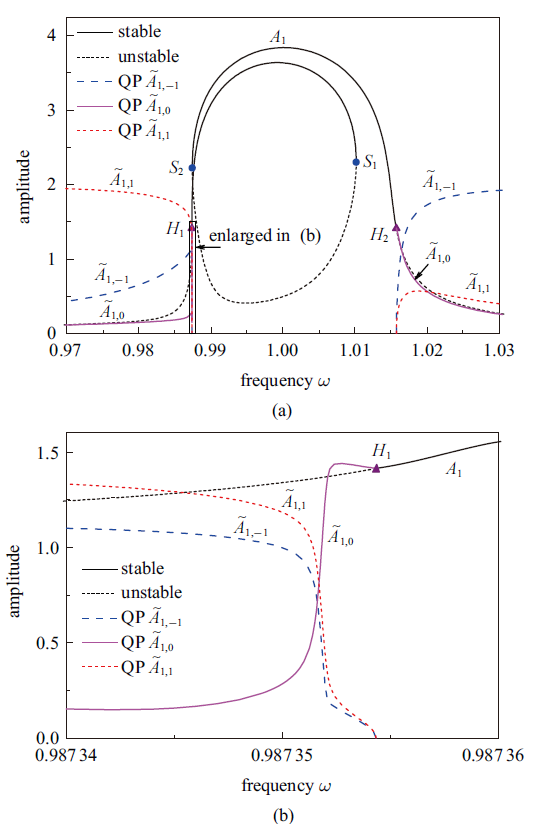Fig.3   Frequency response curves $\omega$ -$A_1$ of periodic response and $\omega$ -$\tilde{A}_{1,-1}$, $\omega$ -$\tilde{A}_{1,0}$, and $\omega$ -$\tilde{A}_{1,1}$ of quasi-periodic (QP) motion of the van der Pol-Mathieu equation with external excitation with $k_1=0.01$, $k_2=0.01$, $k_3=0.05$, and $f=0.01$, (b) is an enlarged view of a zone highlighted in (a)

### 图4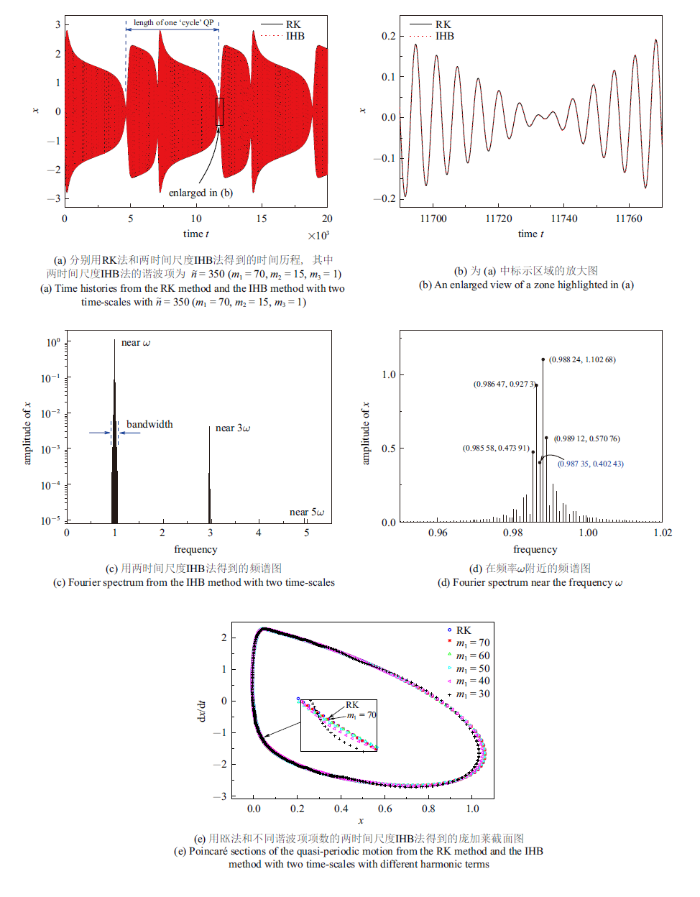Fig.4   Quasi-periodic motion of the van der Pol-Mathieu equation with external excitation with $k_1=0.01$, $k_2=0.01$, $k_3=0.05$, and $f=0.01$ at the freqeuncy $\omega=0.987 35$ near the bifurcation point $H_1$

### 图5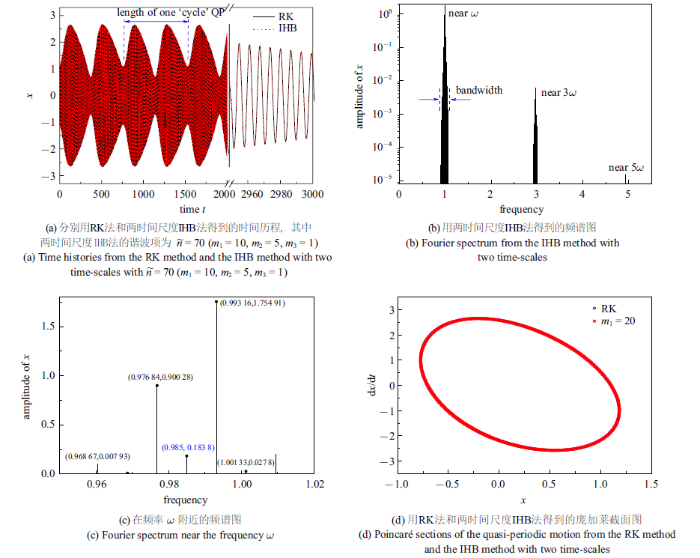Fig.5   Quasi-periodic motion of the van der Pol-Mathieu equation with external excitation with $k_1=0.01$, $k_2=0.01$, $k_3=0.05$, and $f=0.01$ at the parametric excitation freqeuncy $\omega=0.985$

### 图6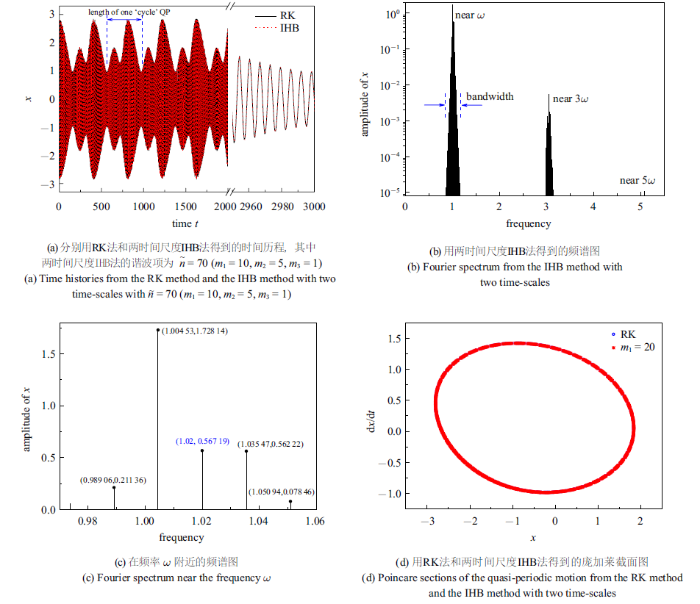Fig.6   Quasi-periodic motion of the van der Pol-Mathieu equation with external excitation with $k_1=0.01$, $k_2=0.01$, $k_3=0.05$, and $f=0.01$ at the freqeuncy $\omega=1.020$}

Table 2  A prior unknown $\omega_{\rm d}$ and three amplitudes $\tilde{A}_{1,-1}$, $\tilde{A}_{1,0}$, and $\tilde{A}_{1,1}$ in frequency response curves of the van der Pol-Mathieu equation with external excitation that are calculated by the IHB method with two time-scales at the three points $\omega=0.987 35,0.985,1.02$ in Fig.3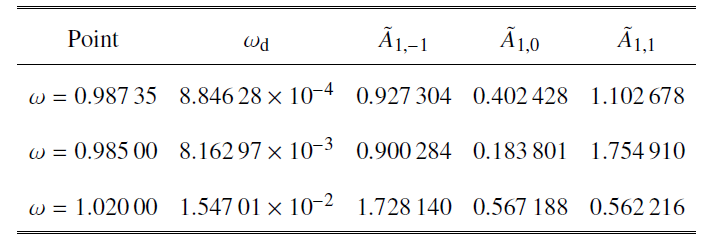Table 3  Comparison of $A_{\text{max}}$ and $A_{\text{min}}$ from the RK method and the IHB method with two time-scales with different harmonic terms at the point $\omega=0.987 35$, where $A_{\text{max}}$ and $A_{\text{min}}$ are the maximal and minimal value of envelops of the quasi-periodic motions, respectively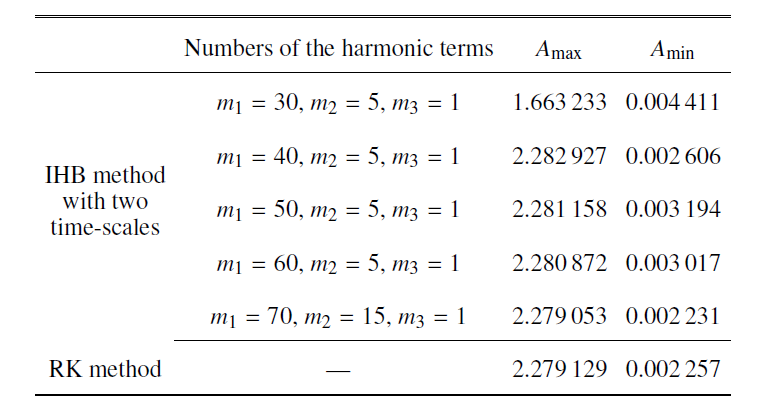## 3 结论

(2) 含外激励van der Pol-Mathieu方程中, 由于自激振动和参数激振联合作用下, 会产生准周期运动响应, 并发现其新特性. 在准周期运动的频谱中,频率$\omega, 3\omega, 5\omega$附近含有边频带, 且边频带是均匀的,即边频带中相邻频率的间距$\omega_{\rm d}$是一样的;在准周期运动的时间历程图中,准周期的包络线也有周期', 其周期'为$2\pi\omega/\omega_{\rm d}$.

(3) 在不同点上的准周期运动响应有不同的动力学特征. 越靠近Hopf分岔点的准周期运动响应, 其边频带的带宽越小, 且其频率分布越密集, 同时其包络线的`周期'越长. 另外, 在不同点上的准周期运动响应所含的频率数目也不同.

## 参考文献 原文顺序 文献年度倒序 文中引用次数倒序 被引期刊影响因子

Iwatsubo T, Saigo M.

Transverse vibration of a rotor system driven by a cardan joint

Journal of Sound and Vibration, 1984,95(1):9-18

Doi M, Masuko I, Ito Y. et al.

A study on parametric vibration in chuck work

Bulletin of JSME, 1985,28(245):2774-2780

Warminski J, Litak G, Szabelski K.

Synchronisation and chaos in a parametrically and self-excited system with two degrees of freedom

Nonlinear Dynamics, 2000,22(2):125-143

Momeni M, Kourakis I, Moslehi-Fard M. et al.

A van der Pol-Mathieu equation for the dynamics of dust grain charge in dusty plasmas,

Journal of Physics A: Mathematical and Theoreitcal, 2007,40(24):F473-F481

Belhaq M, Kirrou I, Mokni L.

Periodic and quasiperiodic galloping of a wind-excited tower under external excitation

Nonlinear Dynamics, 2013,74(3):849-867

Kirrou I, Mokni L, Belhaq M.

On the quasiperiodic galloping of a wind-excited tower

Journal of Sound and Vibration, 2013,332(18):4059-4066

Tondl A.

On the interaction between self-excited and parametric vibrations. National Research Institute for Machine Design, Monographs and Memoranda No. 25,

Prague 1978

Kotera T, Yano S.

Periodic solutions and the stability in a non-linear parametric excitation system

Bulletin of JSME, 1985,28(241):1473-1480

Van der pol-Duffing-Mathieu型系统主参数共振分岔解的普适分类

( Chen Yushu, Xu Jian.

Van der Pol type-Duffing-Mathieu system primary parameter resonance bifurcation solution of the general classfication

Science in China A. 1995,25(12):1287-1297 (in Chinese))

Szabelski K, Warminski J.

Self-excited system vibrations with parametric and external excitation

Journal of Sound and Vibration, 1995,187(4):595-607

( Peng Xian, Chen Zili.

Asymptotic analysis for resonance cycle solution of a type of strongly nonlinear systems

Journal of Dynamics and Control. 2004,2(1):46-50 (in Chinese))

Belhaq M, Fahsi A.

2:1 and 1:1 frequency-locking in fast excited van der Pol-Mathieu-Duffing oscillator

Nonlinear Dynamics, 2007,53(1-2):139-152

Pandey M, Rand RH, Zehnder AT.

Frequency locking in a forced Mathieu-van der Pol-Duffing system

Nonlinear Dynamics, 2007,54(1-2):3-12

( Zhang Qichang, Feng Jingjing, Wang Wei.

The construction of homoclinic and heteroclinic orbit in two-dimentsional nonlinear systems based on the quasi-Padé approximation,

Chinese Journal of Theoretical and Applied Mechanics, 2011,43(5):914-921 (in Chinese))

Warminski J.

Nonlinear dynamics of self-, parametric, and externally excited oscillator with time delay: van der Pol versus Rayleigh models

Nonlinear Dynamics, 2019,99(1):35-56

( Wang Yanqing, Guo Xinghui, Liang Hongkun, et al.

Nonlinear vibratory characteristics and bifurcations of shrouded blades

Chinese Journal of Theoretical and Applied Mechanics. 2011,43(4):755-764 (in Chinese))

( Zhang Dengbo, Tang Youqi, Chen Liqun.

Nonlinear vibration of axially moving beams with nonhomogeneous boundary conditions

Chinese Journal of Theoretical and Applied Mechanics. 2019,51(1):218-227 (in Chinese))

2:1 内共振条件下变转速预变形叶片的非线性动力学响应

( Gu Wei, Zhang Bo, Ding Hu, et al.

Nonlinear dynamic response of pre-deformation blade with variable rotational speed under 2:1 internal resonance

Chinese Journal of, Theoretical and Applied Mechanics, 2020,52(4):1131-1142 (in Chinese))

Duffing 型系统的不动点混沌和 Fold/Fold 簇发现象及机理分析

( Chen Yani, Meng Wenjing, Qian Youhua.

Fixed point chaos and Fold/Fold bursting of a class of Duffing systems and the mechanism analysis

Chinese Journal of Theoretical and Applied Mechanics. 2020,52(5):1475-1484 (in Chinese))

Nayfeh AH, Mook DT.

Nonlinear Oscillations

Wiley, 1995

Belhaq M, Houssni M.

Quasi-periodic oscillations, chaos and suppression of chaos in a nonlinear oscillator driven by parametric and external excitations

Nonlinear Dynamics, 1999,18(1):1-24

Abouhazim N, Belhaq M, Lakrad F.

Three-period quasi-periodic solutions in the self-excited quasi-periodic Mathieu oscillator

Nonlinear Dynamics, 2005,39(4):395-409

Fan Q, Leung AYT, Lee YY.

Periodic and quasi-periodic responses of van der Pol-Mathieu system subject to various excitation

International Journal of Nonlinear Sciences and Numerical Simulation, 2016,17(1):29-40

Veerman F, Verhulst F.

Quasiperiodic phenomena in the van der Pol-Mathieu equation

Journal of Sound and Vibration, 2009,326(1-2):314-320

Huang JL, Zhu WD.

An incremental harmonic balance method with two timescales for quasiperiodic motion of nonlinear systems whose spectrum contains uniformly spaced sideband frequencies

Nonlinear Dynamics, 2017,90(2):1015-1033

Huang JL, Zhu WD.

A new incremental harmonic balance method with two time scales for quasi-periodic motions of an axially moving beam with internal resonance under single-tone external excitation

Journal of Vibration and Acoustics, 2017,139(2):021010

Huang JL, Zhou WJ, Zhu WD.

Quasi-periodic motions of high-dimensional nonlinear models of a translating beam with a stationary load subsystem under harmonic boundary excitation

Journal of Sound and Vibration, 2019,462:114870

Cheung YK, Chen SH, Lau SL.

Application of the incremental harmonic balance method to cubic non-linearity systems

Journal of Sound and Vibration, 1990,140(2):273-286

( Chen Shuhui. Quantitative Analysis Methods for Strongly Nonlinear Vibration. Beijing: Science Press, 2007 (in Chinese))

Hsu CS.

Impulsive parametric excitation: Theory

Journal of Applied Mechanics, 1972,39(2):551-558

Hsu CS.

On approximating a general linear periodic systems

Journal of Mathematical Analysis and Applications, 1974,45(1):234-251

Hsu CS, Cheng WH.

Applications of the theory of impulsive parametric excitation and new treatments of general parametric excitation problems

Journal of Applied Mechanics, 1973,40(1):78-86

Friedmann P, Hammond CE, Woo TH.

Efficient numerical treatment of periodic systems with application to stability problems

International Journal of Numerical Methods in Engineering, 1977,11(7):1117-1136

Huang JL, Su RKL, Chen SH.

Precise Hsu's method for analyzing the stability of periodic solutions of multi-degrees-of-freedom systems with cubic nonlinearity

Computers and Structures, 2009,87(23-24):1624-1630

Zhong WX, Williams FW.

A precise time step integration method

Proceedings of the Institution of Mechanical Engineers, Part C: Journal of Mechanical Engineering Science, 1994,208(6):427-430

/

 〈〉